Algebra 1 : Whole and Part

Example Questions

Example Question #61 : Whole And Part

What number is forty percent of thirty five?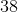Explanation:

A percentage is some number out of 100.  Set up an equation to find the missing number.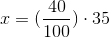Simplify this equation.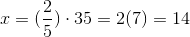The answer is:Example Question #62 : Whole And Part

What is thirty percent of 90?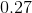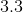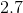Explanation:

Rewrite 30 percent into a fraction.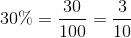Multiply this with 90.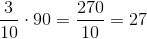The answer is.

Example Question #63 : Whole And Part

What is 76% of 24?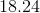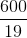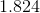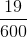Explanation:

To find the value, we must convert the percent to a fraction:

76%=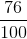.

Now, because "of" indicates multiplication, we multiply the fraction by the "whole":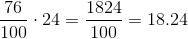Example Question #64 : Whole And Part

What is 60 percent of 99?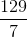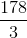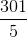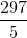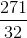Explanation:

In order to determine the part, we will need to multiply the percent with the whole.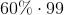Convert the percent to a fraction.  Remove the percentage sign and divide by 100.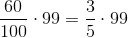Multiply the numerator with 99.

The exact answer is:Example Question #65 : Whole And Part

What is 40 percent of one-fourth?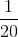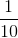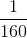Explanation:

Rewrite the given percentage into a fraction.  A percentage is some number out of 100 parts.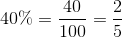Multiply this fraction by one-fourth.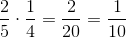The answer is:Example Question #66 : Whole And Part

Find the number that is sixty percent of forty.Explanation:

Write sixty percent of forty as an expression.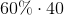The percentage can be converted to a fraction by removing the percentage sign and divide by 100.

Rewrite the expression and simplify.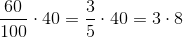The answer is:Example Question #67 : Whole And Part

What is forty percent of eighty?Explanation:

A percent is some number out of 100 parts.  Rewrite 40 percent into a fraction.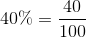Write the expression for forty percent of eighty.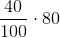Simplify the expression.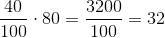The answer is:Example Question #68 : Whole And Part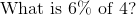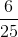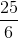Explanation:

Rewrite the percentage as a fraction. A percent is some number out of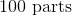.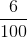Multiply this fraction by.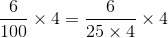Cancel out the common terms in the denominator with the integer.

The answer is:Example Question #69 : Whole And Part

What is three percent of three?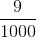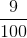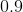Explanation:

Rewrite three percent as a fraction.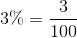Multiply this fraction with three.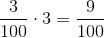The answer is:Example Question #70 : Whole And Part

What is four percent of twelve?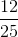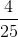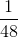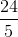Explanation:

Write four percent as a fraction.  A percent is a number out of 100 parts.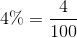Multiply this fraction with twelve.Reduce this fraction.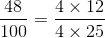Cancel the common terms of this fraction.

The answer is: